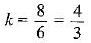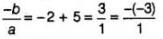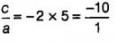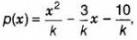# MCQ : Polynomials - 1

## 10 Questions MCQ Test Mathematics (Maths) Class 10 | MCQ : Polynomials - 1

Description
This mock test of MCQ : Polynomials - 1 for Class 10 helps you for every Class 10 entrance exam. This contains 10 Multiple Choice Questions for Class 10 MCQ : Polynomials - 1 (mcq) to study with solutions a complete question bank. The solved questions answers in this MCQ : Polynomials - 1 quiz give you a good mix of easy questions and tough questions. Class 10 students definitely take this MCQ : Polynomials - 1 exercise for a better result in the exam. You can find other MCQ : Polynomials - 1 extra questions, long questions & short questions for Class 10 on EduRev as well by searching above.
QUESTION: 1

### If 5 is a zero of the quadratic polynomial, x2 - kx - 15 then the value of k is

Solution:

x=5

putting x in equation

p(x)=x2-kx-15=0

=(5)2-k(5)-15=0

=25-5k-15=0

=10-5k=0

=-5k=-10

=k=-10/-5

=k=2

Value of k is 2

QUESTION: 2

### If p(x) is a polynomial of at least degree one and p(k) = 0, then k is known as

Solution:

Zero of p(x)
Let p(x) = ax + b
Put x = k
p(k) = ak + b = 0
∴ k is zero of p(x)

QUESTION: 3

### The zero of the polynomial p(x) = 2x + 5 is

Solution:

Given, p(x) = 2x+5
For zero of the polynomial, put p(x) = 0
∴ 2x + 5 = 0
⇒ -5/2

Hence, zero of the polynomial p(x) is -5/2.

QUESTION: 4

If one of the zeroes of the quadratic polynomial (k - 1)x2 + kx + 1 is -3, then the value of k is

Solution:

(k - l)x2 + kx +1

One zero is - 3, so it must satisfy the equation and make it zero
∴ (k - 1) (-3)2 + k(-3) + 1 - 0
⇒ 9k - 9 - 3k + 1 = 0
⇒ 6k - 8 = 0
⇒QUESTION: 5

If one of the zeros of a quadratic polynomial of the form x2 + ax + b is the negative of the other, then it

Solution:

Let p(x) = x2 + ax + b.
Put a = 0, then,  p(x) = x2 + b = 0
⇒    x= -b
⇒  x =  ±-b
[∴b < 0]
Hence, if one of the zeroes of quadratic polynomial p(x) is the negative of the other, then it has no linear term i.e., a = 0 and the constant term is negative i.e., b< 0.
Alternate Method
Let   f(x) = x2 + ax+ b
and by given condition the zeroes area and – α.
Sum of the zeroes = α- α = a
= a = 0
f(x) = x2 + b, which cannot be linear,
and product of zeroes = α .(- α) = b
⇒ -α2 = b
which is possible when, b < 0.
Hence, it has no linear term and the constant term is negative.

QUESTION: 6

If the zeroes of the quadratic polynomial x2 + (a + 1) x + b are 2 and -3, then

Solution:

x2 + (a + 1)x + b
∵  x = 2 is a zero and x = - 3 is another zero
∴ (2)2 + (a + 1)2 + b = 0
and (-3)2 + (a + 1) (-3) + b = 0
⇒ 4 + 2a + 2 + b = 0 and 9 - 3a - 3 + b = 0
⇒ 2a + b = -6 ...(i) and -3a + b = -6 ..(ii)
Solving (i) and (ii), we get 5a = 0
⇒ a = 0 and b = -6.

QUESTION: 7

The number of polynomials having zeros as - 2 and 5 is

Solution:

Let p (x) = ax2 + bx + c be the required polynomial whose zeroes are -2 and 5.

∴ Sum of zeroes = -b/a
⇒...(i)
and product of zeroes = c/a
⇒...(ii)
From Eqs. (i) and (ii)
a = 1, b = -3 and c = -10
∴ p(x) = ax2 + bx + c = 1.x2 - 3x - 10
= x2 - 3x - 10
But we know that, if we multiply/divide any polynomial by any arbitrary constant. Then, the zeroes of polynomial never change.
∴ p(x) = kx2 - 3kx - 10k [where, k is a real number]
⇒[where, k is a non-zero real number]

Hence, the required number of polynomials are infinite i.e., more than 3.

QUESTION: 8

Which of the following is NOT the graph of a quadratic polynomial ?

Solution:

For any quadratic polynomial ax2 + bx + c, a≠0, the graph of the Corresponding equation y = ax2 + bx + c has one of the two shapes either open upwards like u or open downwards like  ∩ depending on whether a > 0 or a < 0. These curves are called parabolas.
Also, the curve of a quadratic polynomial crosses the X-axis on at most two points but in option (a) the curve crosses the X-axis on the three points, so it does not represent the quadratic polynomial.

QUESTION: 9

If one root of the polynomial p(y) = 5y2 + 13y + m is reciprocal of other, then the value of m is

Solution:

Let the roots be α and 1/α. Then α(1/α) = m/5 or 1 = m/5 or m = 5

QUESTION: 10

If p(x) = ax2 + bx + c, then - b/a is equal to

Solution:

Sum of zeroes = -b/a